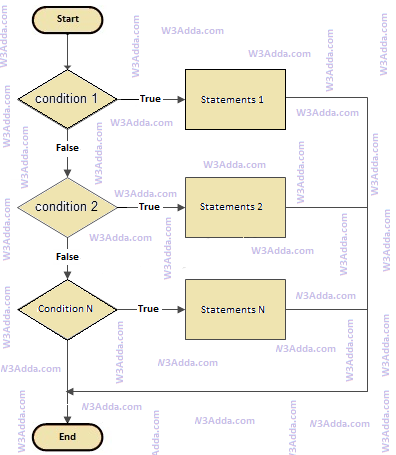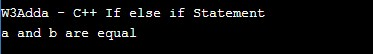# C++ if else if Statement

In this tutorial you will learn about the C++ if else if Statement and its application with practical example.

## C++ if else if Statement

In C++, if..else..if statement allows us add alternative set of test conditions in if..else statement using else-if and single else statements for if condition. In such way if..else..if statement is used to select one among several blocks of code to be executed.

## C++ if..else..if Statement Flow Diagramcplusplus-if-else-if

Syntax:-

Example:-

Output:-In this tutorial we have learn about the C++ if else if Statement and its application with practical example. I hope you will like this tutorial.